# Falsifying Stock-to-Flow As a Model of Bitcoin Value

## The Drunken Value of Bitcoin

— — -BEGIN BITCOIN SIGNED MESSAGE — — -
Falsifying Stock-to-Flow As a Model of Bitcoin Value
— — -BEGIN SIGNATURE — — -
IFk9sASioI2p8nMVAuJ0VFoQdU99YF63JrterSNnBaYKd648KTvmom9gaPiZwnu9b/22/ZrKpf1AB75saMPBphs=
— — -END BITCOIN SIGNED MESSAGE — — -

# Abstract

This article explores if there is a stock-to-flow relationship to Bitcoin value. The proposed log-log model is tested for statistical validity against the least squares assumptions, for stationarity in each variable and for potential spurious relationships. A Vector Error Correction Model (VECM) is built and tested against the original stock-to-flow model. Whilst some of these models out-compete the original model in terms of Akaike Information Criteria, all of them fail to reject the hypothesis that stock-to-flow is an important non-spurious predictor for the value of Bitcoin.

# Notes

• All analysis was performed using Stata 14.

# Introduction

Scientific method is difficult for most to comprehend. It is counterintuitive. It can lead to conclusions that do not reflect personal beliefs. It takes a foundation in the method to understand this basic fundamental concept: it is ok to be wrong. This should be something that is taught in school. If we are afraid of getting it wrong, we will never propose anything new. The history of scientific discovery is therefore by its’ very nature surrounded in serendipity. Things that people discover by accident can be just as important as (or more important than) whatever it is they originally set out to do. Their original ideas might have been incorrect or inconclusive, but the things they discovered on the journey built the framework for those who follow.

According to the great modern scientific philosopher Karl Popper, testing a hypothesis for an incorrect outcome is the only reliable way to add weight to the argument that it is correct¹. If rigorous and repeated tests cannot show that a hypothesis is incorrect, then with each test the hypothesis assumes a higher likelihood of being correct. This concept is called Falsifiability. This article aims to falsify the stock-to-flow model of Bitcoin value, as defined in Modelling Bitcoin’s Value with Scarcity².

# Defining The Problem

To falsify a hypothesis, first we must state what it is:

Null Hypotheses (H0): The value of Bitcoin is a function of the stock-to-flow of Bitcoin

Alternative Hypotheses (H1): The value of Bitcoin is not a function of the stock-to-flow of Bitcoin

The author of  chose to test H0 by fitting an Ordinary Least Squares (OLS) regression on the natural log of the market capitalization of Bitcoin and the natural log of the stock-to-flow. There was no accompanying diagnostics nor any identified reasoning for the log transformation in both variables, other than the idea that a log-log model can be expressed as a power law. The model did not take into account the possibility of a spurious relationship due to non-stationarity.

# Approach

In this article, we will explore that model and run it through the normal regression diagnostics and determine if the log transformation was necessary or appropriate (or both) and explore possible confounding variables, interactions, and sensitivity.

Another issue that will be explored is that of non-stationarity. Stationarity is an assumption of most statistical models. It is the concept that through time, there is no trend in any moments, for example, if there is no trend in the mean (or variance) with respect to time.

Consequent to the stationarity analysis, we will explore the possibility of cointegration.

# Notation

Medium is relatively limited for mathematical notation. The usual notation for an estimate of a statistical parameter is to place a hat on top. Instead, we define the estimate of a term as []. e.g. the estimate of β = [β]. If we are representing a 2x2 matrix, we will do so like this [r1c1, r1c2 \ r2c1, r2c2] etc. Subscripted items are superseded by @ — eg for the 10th position in a vector X we would normally subscript X with 10. We will instead write X@10.

# Ordinary Least Squares

Ordinary least squares regression is a way to estimate a linear relationship between two or more variables.

First, let us define a linear model as some function of X that equals Y with some error.

Y = βX+ε

where Y is the dependent variable, X is the independent variable, ε is the error term and β is the multiplier of X. The goal of OLS is to estimate β such that ε is minimized.

In order for [β] to be a reliable estimate, some basic assumptions must be met:

1. There is a linear relationship between the dependent and independent variables

## Linearity

We begin by taking a look at the non-transformed to scatter plot of market cap v stock-to-flow (data from )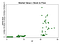Figure 1 — Market cap v stock to flow. The data are too sparse to ascertain a relationship.

In figure 1, we encounter a good reason to take the log of the market value — the span is much too broad. Taking the log of market value (but not SF) and re-plotting gives us a familiar log looking pattern (figure 2).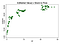Figure 2 — log market cap v SF. A clear logarithmic pattern is arising.

Taking the log of the stock-to-flow and again plotting gives us the obvious linear pattern identified by the author of  in Figure 3.

This confirms the choice of log-log — the only transformations that really show a good linear relationship.

An alternative transformation was taking the square root of both. This pattern is displayed in figure 4.

Clearly, the log-log transformation is the most appropriate to meet the first assumption requirement (linearity).

Thus the preliminary analysis cannot reject H0.

The log-log fitted regression is given in figure 5 below, where [β] = [3.4, 3.7] (95% Confidence Interval)

Using the model, we can now estimate the residuals [ε] and fitted values [Y] and test the other assumptions.

# Homoscedasticity

If the assumption of constant variance in the error term (i.e. homoscedasticity) were to be true, then the error term would vary randomly around 0 for each value in the predicted values. The RVF plot (figure 6) is, therefore, a simple yet effective graphical way to investigate the accuracy of this assumption. In figure 6, we see there is a bit of a pattern, rather than a random scattering, indicating a non-constant variance in the error term (i.e. heteroscedasticity).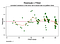Figure 6 — RVF plot. A pattern here indicates there might be an issue.

Heteroscedasticity like this causes the estimates of the coefficients [β] to have a larger variance and thus be less precise and leads to p-values that are more significant than they should be, because the OLS procedure does not detect the increased variance. Therefore when we then calculate t-values and F values we use an underestimation of the variance, leading to higher significance. This also has an effect on the 95% confidence interval about [β], which is itself a function of the variance (via the standard error).

At this stage, it would be safe to continue with the regression understanding that these problems exist. There are ways we can deal with these issues — bootstrapping or using a robust estimator for the variance for example.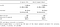Figure 7 — The impact of the heteroscedasticity is shown in the robust estimation

As can be seen in figure 7, whilst there is a small increase in the variance (see the broadened confidence interval), for the most part, the heteroscedasticity present isn’t really having that much of a detrimental effect.

At this stage, we cannot reject H0 due to heteroscedasticity.

## Normality In Error

The assumption that the error term is normally distributed with a mean of zero is a less important assumption to meet than linearity or homoscedasticity. Non-normality, but non-skewed residuals are going to have the effect of making the confidence intervals too optimistic. If the residuals are skewed then you might end up with a little bias. However, as we can see from figures 8 and 9, the residuals are normal enough. The mean is ostensibly zero and whilst a formal test would likely reject the hypothesis of normality, they fit the normal curve enough for the confidence intervals to be unaffected.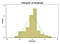Figure 8— histogram of the error term with a normal distribution (green) overlaid.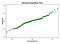Figure 9 — normal quantiles plot of the error term. The closer the dots are to the line the better the normal fit.

## Leverage

Leverage is the concept that not all data points in the regression contribute equally to the estimation of the coefficients. Some points with high leverage could significantly alter the coefficient depending on if they are present or not. In figure 10, we can see quite clearly that there are some concerning points from early on (March, April and May 2010). This is not too surprising and the author of  has stated previously that there was some concern with gathering the earlier values.

If we re-run the regression without those points (let's assume there is some mistake in them) and since we know about the heteroscedasticity problem, we should use the robust estimator.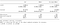Figure 11 — Removal of the high leverage points has substantially changed the estimation of [β], and improved the Akaike information criteria (AIC).

In figure 11 we can see that by removing these three points, the estimation of [β] is substantially different, and the Akaike Information Criteria is substantially lower, indicating that the model is a better model, despite the lower R².

## Ols Summary

Basic diagnostics indicate a few small fixable problems with the original OLS. We cannot at this stage reject H0.

# Stationarity

A stationary process is said to be Order 0 integrated (eg I(0)). A non-stationary process is I(1) or more. Integration in this context is more like a poor-mans integration — it is the sum of the lagged difference. I(1) means that if we subtract the first lag from each value in the series, we will have an I(0) process. It is relatively well known that regression on non-stationary time-series can lead to the identification of spurious relationships.

In figures 12 & 13 below, we can see that we cannot reject the null hypothesis of the ADF test. The null hypothesis of the ADF test is that the data are non-stationary. This means we cannot say that the data are stationary.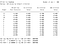Figures 12 & 13 — GLS Augmented Dickey Fuller tests for unit root on ln(Market Value) and ln(SF).

The Kwiatkowski-Phillips-Schmidt-Shin test is a complimentary test for stationarity to the ADF tests. This test (KPSS) has the null hypothesis that the data are stationary. As we can see in figures 14 & 15, we can reject stationarity for most lags in both variables.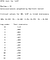Figures 14 & 15 — KPSS test against the null of stationarity

These tests prove that these two series beyond any doubt are non-stationary. This is a bit of a problem. If the series isn’t at least trend stationary then OLS could be misguided into identifying a spurious relationship. One thing we could do is take the log-monthly difference of each variable and rebuild our OLS. However; thanks to this issue being rather common in econometric series, there is a much more robust framework available to us — something called cointegration.

# Cointegration

Cointegration is a way to deal with a pair (or more) of I(1) processes and determine if there is a relationship and what that relationship is. To understand cointegration we give a simplified example of a drunk and her dog . Imagine a drunk walking her dog home on a leash. The drunk is walking all over the place, unpredictably. The dog walks pretty randomly as well: sniffing trees, barking, chasing scratching — just generally being a mutt. However, the dog’s overall direction will be within the length of the leash of the drunk. We could estimate that for any point on the drunks walk home, the dog will be within leash length of the drunk (sure it might be on one side or another, but the dog will be within leash length). This bad simplification is a rough metaphor of cointegration — the dog and the owner are moving together.

Contrast this to correlation — Let's say a stray dog follows the drunk’s mangy mongrel for 95% of the way home and then runs off to chase a car to the other side of town. There would be a very strong correlation between the path of the stray and the drunk (literally R²: 95%), however much like the many one night stands that the drunk has had — that relationship didn’t mean anything — it can’t be used to predict where the drunk will be as whilst for some part of the trip it is true, for some parts it is wildly inaccurate.

In order to find the drunk, first, we will see what lag-order specification our model should use.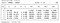Figure 16 — Lag order specification. Minimum AIC is used to determine.

We identify here the most appropriate lag-order to investigate via the selection of the minimum AIC is an order of 2.

Next, we need to identify if there is a cointegrating relationship. The Johansen framework [5,6,7] gives us excellent tools to do this with.

The results in figure 17 gives us evidence to say there is at least 1 cointegrating equation between lnvalue and lnSF.

We define our VECM as:

Δy@t =αβ`y@t-1+Σ(Γ@iΔy@t-1)+v+δt+ε@t

In the figures above, we have estimated:

• [α] = [-0.14, 0.03]

Overall, the output indicates that the model fits well. The coefficient on ln(SF) in the cointegrating equation is statistically significant, as are the adjustment parameters. The adjustment parameters indicate that when predictions from the cointegrating equation are positive, ln(value) is below its equilibrium value because the coefficient on ln(value) in the cointegrating equation is negative. The estimate of the coefficient [D lnvalue]L. ce1 is -0.14.

Thus when the Bitcoin value is too low, it quickly rises back toward the lnSF fit. The estimated coefficient [D lnSF]L. ce1 of 0.028 implies that when the Bitcoin value is too low, it adjusts towards the equilibrium.

In the figure above we can see that there is a tendency towards zero for the cointegrating equation. Whilst it may not be formally stationary it is definitely approaching stationarity.

From the STATA manual:

The companion matrix of a VECM with K endogenous variables and r cointegrating equations has K􀀀r unit eigenvalues. If the process is stable, the moduli of the remaining r eigenvalues are strictly less than one. Because there is no general distribution for the moduli of the eigenvalues, ascertaining whether the moduli are too close to one can be difficult.

The graph of the eigenvalues shows that none of the remaining eigenvalues appear close to the unit circle. The stability check does not indicate that our model is misspecified.

The figure above indicates that an orthogonalized shock to the stock-to-flow value has a permanent effect on the value of Bitcoin.

And here is where we draw the line. Stock-to-flow is not a random variable. It is a function with a known value over time. There won't ever be a shock to stock-to-flow — its value can be calculated ahead of time with precision. However, this model provides very strong evidence that there is a fundamental non-spurious relationship between stock-to-flow and Bitcoin value.

# Limitations

In this study, we did not account for any confounding variables. Given the evidence above it is unlikely that any confounders would have a significant impact to our conclusion — we cannot reject H0. We cannot say “there is no relationship between stock-to-flow and Bitcoin value”. If that were the case, then there would be no cointegrating equation.

# Conclusion

Whilst some of the models presented here out-compete the original model in terms of Akaike Information Criteria, all of them fail to reject the hypothesis that stock-to-flow is an important non-spurious predictor for the value of Bitcoin.

To illustrate this with a metaphor: if we consider the value of bitcoin as the drunk then the stock-to-flow is not really the dog she walks — it is more like the road she walks on. The drunk will wander all over the road, sometimes stopping, slipping up and missing a turn here and there or even taking short cuts along the way; but generally, she will follow the road home.

In short, Bitcoin is the drunk and Stock-to-Flow is the road home.

# Citations

1. Popper, Karl (1959). The Logic of Scientific Discovery (2002 pbk; 2005 ebook ed.). Routledge. ISBN 978–0–415–27844–7.